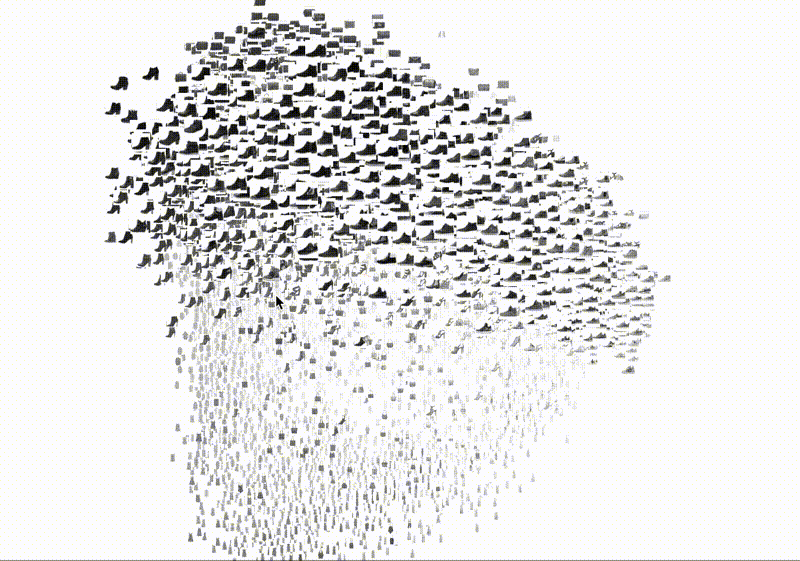# Classify Images of Clothing using Tensorflow

Original article was published on Deep Learning on MediumTensorFlow works based on data flow graphs that have nodes and edges. It accepts data in the form Tensors, which are nothing but multi-dimensional arrays of higher dimensions and can handle large amounts of data.

# 1. Import important Libraries

We will be using tf.keras, which is TensorFlow’s high-level API for building and training deep learning models. It’s used for fast prototyping and create new layers, metrics, loss functions, and develop state-of-the-art models.

Numpy is a core library for scientific computing in Python and Matplotlib is a library for creating static, animated, and interactive visualizations.

`# import TensorFlow, tf.keras, numpy and matplotlibimport tensorflow as tffrom tensorflow import kerasimport numpy as npimport matplotlib.pyplot as plt`

# 2. Import the dataset

We will be using Fashion MNIST dataset in this project. The image contains 70,000 grayscale images in 10 categories, out of which 60,000 images are used to train the network and 10,000 images to evaluate how accurately the network learned to classify images.

`fashion_mnist = keras.datasets.fashion_mnist#returns four numpy 28x28 arrays with pixel values ranging from 0 to #255(train_images, train_labels), (test_images, test_labels) = fashion_mnist.load_data()`

Each image is mapped to a single label. Labels are nothing but the array of integers, ranging from 0 to 9 and correspond to the class of the clothing the image represents.

Training sets represents the data that the model uses to learn — `train_images` and `train_labels` arrays.

Test sets represent the data that the model uses to test — `test_images`, and `test_labels` arrays.

`class_names = ['T-shirt/top', 'Trouser', 'Pullover', 'Dress',  'Coat','Sandal', 'Shirt', 'Sneaker', 'Bag', 'Ankle  boot']`

# 3. Data Exploration

`train_images.shape`

(60000, 28, 28)

`len(train_labels)`

60000

`test_images.shape`

(10000, 28, 28)

`len(test_labels)`

10000

# 4. Data Preprocessing

For both training and test sets, you can see that the pixel values of the images fall in the range of 0 to 255, which needs to be scaled in the range of 0 and 1 before feeding it in the neural network. To do so, divide the values by 255.

`train_images = train_images / 255.0test_images = test_images / 255.0`

To verify that the data is in the correct format, display the first 25 images from the training set, and display the class name below each image.

`plt.figure(figsize=(10,10))for i in range(25): plt.subplot(5,5,i+1) plt.xticks([]) plt.yticks([]) plt.grid(False) plt.imshow(train_images[i], cmap=plt.cm.binary) plt.xlabel(class_names[train_labels[i]])plt.show()`